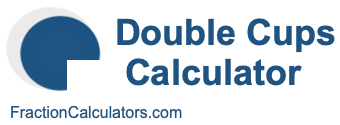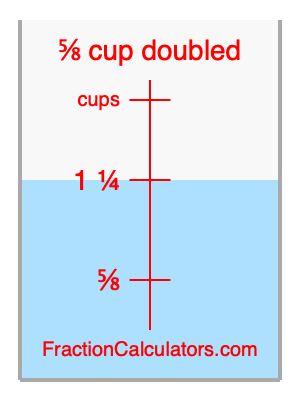What is 5/8 cup doubled?Here we will calculate 5/8 cup doubled and illustrate 5/8 cup doubled on a measuring cup. What is 5/8 cup doubled? 5/8 cup doubled is twice 5/8 cup. Therefore, to double 5/8 cup, we multiply 5/8 by 2. Here is the formula, the math, and the answer to 5/8 cup doubled:

Cup × 2 = Cup doubled
5/8 × 2 = 1 1/4
5/8 cup doubled = 1 1/4 cups

Below is an illustration of a measuring cup. We show 5/8 cup and 5/8 cup doubled (1 1/4 cups) so you can see where they are in relation to each other.As you can see, we filled up our measuring cup to 5/8 cup doubled. In other words, we filled it up to 1 1/4, which is two times 5/8.

Double Cups Calculator
Here you can double another cup measurement.

What is cups doubled?

What is 2/3 doubled?
Here is the next measurement on our list that we have doubled for you.